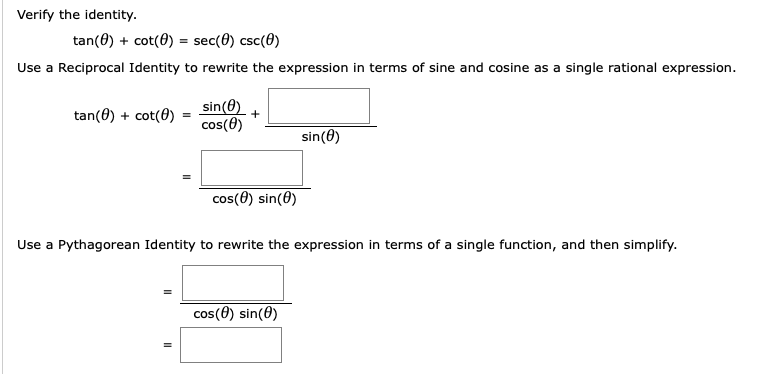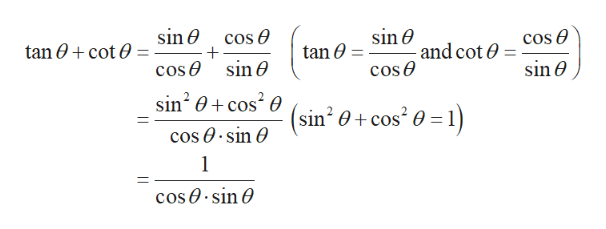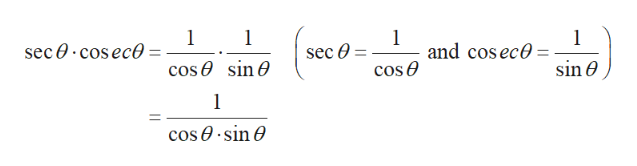# Verify the identity.tan(0) + cot(0) = sec(0) csc(0)Use a Reciprocal Identity to rewrite the expression in terms of sine and cosine as a single rational expression.sin(0)cos(0)tan(0) + cot(0)%3Dsin(0)cos(0) sin(0)Use a Pythagorean Identity to rewrite the expression in terms of a single function, and then simplify.cos(0) sin(0)

Question
6 viewshelp_outlineImage TranscriptioncloseVerify the identity. tan(0) + cot(0) = sec(0) csc(0) Use a Reciprocal Identity to rewrite the expression in terms of sine and cosine as a single rational expression. sin(0) cos(0) tan(0) + cot(0) %3D sin(0) cos(0) sin(0) Use a Pythagorean Identity to rewrite the expression in terms of a single function, and then simplify. cos(0) sin(0) fullscreen
check_circle

Step 1

In order to verify the given expression, first solve the right-hand side, which is given below.help_outlineImage Transcriptionclosesin e - and cot 0 cose sin e cos e cos O tan 0 + cot 0 tan 0 sin e sin e cose sin? 0+ cos? 0 (sin? e+ cos? 0 = 1) cos 0· sin O cos 0· sin O fullscreen
Step 2

Now solve the left-hand side,...help_outlineImage Transcriptionclose1 sec 0· cosece and cos ece cos e sec O cos e sin e sin 0 cos 0· sin O fullscreen

### Want to see the full answer?

See Solution

#### Want to see this answer and more?

Solutions are written by subject experts who are available 24/7. Questions are typically answered within 1 hour.*

See Solution
*Response times may vary by subject and question.
Tagged in

### Calculus